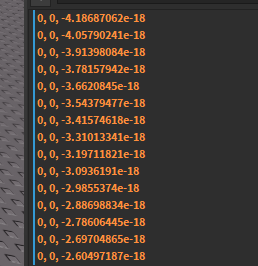# Improving Movement

Hello,
I wanted to know if there’s a way to make Vector3:Lerp() actually reach 0 instead of getting infinitely small. Here an exampleI’m working on a custom character where I’m applying acceleration and this is the result after that calculation. It works I just wanted to know if there was a way to make the lerp function reach 0. Seems like a floating point inaccuracy thing but yea

You’re right, it is due to float precision. The common programmer method to solve this is just to clamp or round it. Just round your values to the nearest thousandth.

``````function roundV3(v3: Vector3)
return Vector3.new(
math.floor(v3.x * 1000) / 1000,
math.floor(v3.y * 1000) / 1000,
math.floor(v3.z * 1000) / 1000
)
end

local val: Vector3 = Vector3.new():Lerp(Vector3.new())
local val_rounded: Vector3 = roundV3(val)
``````
2 Likes